## Cutting Speed(vc)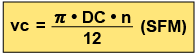vc(SFM):Cutting Speed
DC(inch);Cutter Diameter
π(3.14):Pi
n(min-1):Main Axis Spindle Speed

DC(inch)
n(min-1)
vc(SFM)

### Problem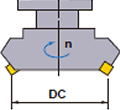What is the cutting speed when main axis spindle speed is 350min-1 and the cutter diameter is ø5" ?

Substitute π=3.14, DC=5", n=350 into the formulae.
vc=π×DC×n÷12=3.14×5"×350÷12=457.9SFM
The cutting speed is 457.9SFM.

## Feed Per Tooth(fz)

Check the item you want to calculate, input values in the three boxes, and then press the Calculate button.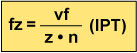vf(IPM):
Table Feed per Min.

z:Insert Number
n(min-1):
Main Axis Spindle Speed (Feed per Revolution f=z
xfz)
fz(IPT):Feed per Tooth

vf(IPM)
z
n(min-1)
fz(IPT)

### Problem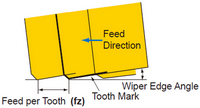What is the feed per tooth when the main axis spindle speed is 500min-1, number of insert is 10, and table feed is 20inch/min?

Substitute the above figures into the formulae.
fz=Vf÷(z×n)=20÷(10×500)=.004IPT

## Table Feed(vf)vf(IPM): Table Feed per Min.
z:Insert Number
n(min-1): Main Axis Spindle Speed
fz(IPT):Feed per Tooth

### ProblemWhat is the table feed when feed per tooth is .004IPT, number of insert is 10, and main axis spindle speed is 500min-1?

Substitute the above figures into the formulae.
vf=fz×z×n=.004IPT×10×500=20inch/min
The table feed is 20inch/min.

## Cutting Time(Tc)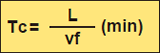L(mm):Total Table Feed Length (Workpiece Length: (l)+Cutter Diameter：DC))
vf(mm/min):Table Feed per Min.
Tc(min):Cutting Time

L(mm)
vf(mm/min)
Tc(min)
second(s)

### Problem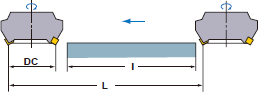What is the cutting time required for finishing 100mm width and 300mm length surface of a cast iron (JIS FC200) block when the cutter diameter is ø200mm, the number of inserts is 16, the cutting speed is 125m/min, and feed per tooth is 0.25mm. (spindle speed is 200min-1

Calculate table feed per min
vf=0.25×16×200=800mm/min
Calculate total table feed length.
L=300+200=500mm
Substitute the above answers into the formula.
Tc=500÷800=0.625(min)
0.625×60=37.5(sec). The answer is 37.5 sec.

## Cutting Speed(vc)

Check the item you want to calculate, input values in the two boxes, and then press the Calculate button.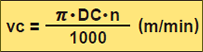*Divide by 1000 to change to m from mm.

DC(mm);Cutter Diameter
π(3.14):Pi
n(min-1):Main Axis Spindle Speed

vc(m/min):Cutting Speed

DC(mm)
n(min-1)
vc(m/min)

### ExampleWhat is the cutting speed when main axis spindle speed is 350min-1 and the cutter diameter is ø125 ?

Substitute π=3.14, DC=125, n=350 into the formula.
vc=(π•DC•n)÷1000=(3.14×125×350)÷1000
=137.4m/min

The cutting speed is 137.4m/min.

## Feed Per Tooth(fz)

Check the item you want to calculate, input values in the three boxes, and then press the Calculate button.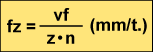vf(mm/min):
Table Feed per Min.

z:Insert Number
n(min-1):
Main Axis Spindle Speed (Feed per Revolution f=z
xfz)
fz(mm/t.):Feed per Tooth

vf(mm/min)
z
n(min-1)
fz(mm/t.)

### ExampleWhat is the feed per tooth when the main axis spindle speed is 500min-1, number of insert is 10, and table feed is 500mm/min?

Substitute the above figures into the formula.
fz=Vf÷(z×n)=500÷(10×500)=0.1mm/t.

## Table Feed(vf)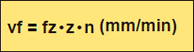vf(mm/min): Table Feed per Min.
z:Insert Number
n(min-1): Main Axis Spindle Speed
fz(mm/t.):Feed per Tooth

### ExampleWhat is the table feed when feed per tooth is 0.1mm/t., number of insert is 10, and main axis spindle speed is 500min-1?

Substitute the above figures into the formula.
vf=fz×z×n=0.1×10×500=500mm/min
The table feed is 500mm/min.

## Cutting Time(Tc)

Input the values.L(mm):Total Table Feed Length (Workpiece Length: (l)+Cutter Diameter：DC))
vf(mm/min):Table Feed per Min.
Tc(min):Cutting Time

L(mm)
vf(mm/min)
Tc(min)
second(s)

### ExampleWhat is the cutting time required for finishing 100mm width and 300mm length surface of a cast iron (JIS FC200) block when the cutter diameter is ø200mm, the number of inserts is 16, the cutting speed is 125m/min, and feed per tooth is 0.25mm. (spindle speed is 200min-1